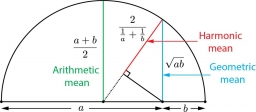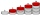# Harmonic mean

If x, y, z form a harmonic progression, then y is the harmonic mean of x and z. Find the harmonic mean of the numbers 6 and 5.

y =  5.4545

### Step-by-step explanation:Did you find an error or inaccuracy? Feel free to write us. Thank you!

Showing 1 comment:Rico
what is −21 ​− 5/3 ​ =Tips to related online calculators
Looking for calculator of harmonic mean?
Looking for a statistical calculator?

## Related math problems and questions:

• Harmonic seriesInsert four members between 5/3 and 5/11 to form harmonic series (means).
• Insert 5Insert five harmonic means between 1/2 and 1/26
• Insert 7Insert five harmonic means between 3 and 18
• Insert 6Insert four harmonic means between 3/7 and 3/19
• HP - harmonic progressionDetermine the 10th term of the harmonic progression 6,4,3,…
• HP - harmonic progressionDetermine the 8th term of the harmonic progression 2, 4/3, 1,…
• Sequence 11What is the nth term of this sequence 1, 1/2, 1/3, 1/4, 1/5?
• Harmonic 4The harmonic mean of -6 and 5.
• HP - harmonic progression 2Compute the 16th term of the HP if the 6th and 11th term of the harmonic progression are 10 and 18 respectively.
• Harmonic meanHarmonic means of 6 and 12
• If the 3If the 6th term of a GP is 4 and the 10th is 4/81, find common ratio r.
• Insert 3Insert five arithmetic progression members between -7 and 3/2.
• PoolIf water flows into the pool by two inlets, fill the whole for 19 hours. The first inlet filled pool 5 hour longer than the second. How long pool take to fill with two inlets separately?
• Find theFind the number x, which if it increases by 2, then its square increases by 21 percent.
• Ratio of sidesThe triangle has a circumference of 21 cm, and the length of its sides is in a ratio of 6: 5: 3. Find the length of the longest side of the triangle in cm.
• Exponential equationFind x, if 625 ^ x = 5 The equation is exponential because the unknown is in the exponential power of 625
• Fractions and mixed numerals(a) Convert the following mixed numbers to improper fractions. i. 3 5/8 ii. 7 7/6 (b) Convert the following improper fraction to mixed number. i. 13/4 ii. 78/5 (c) Simplify these fractions to their lowest terms. i. 36/42 ii. 27/45 2. evaluate following ex